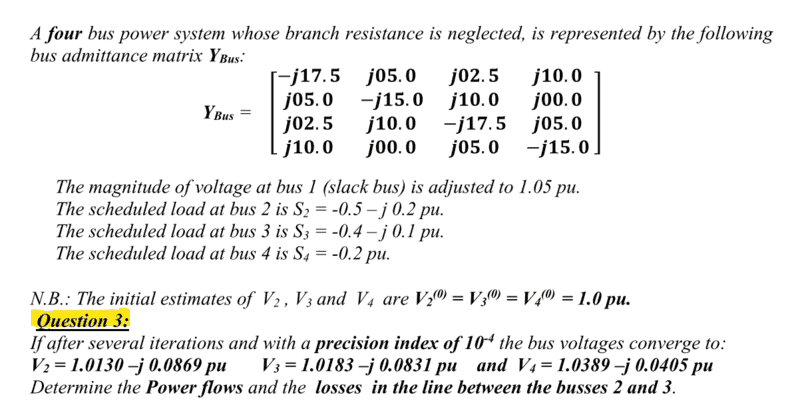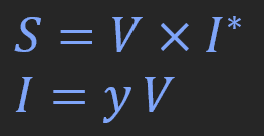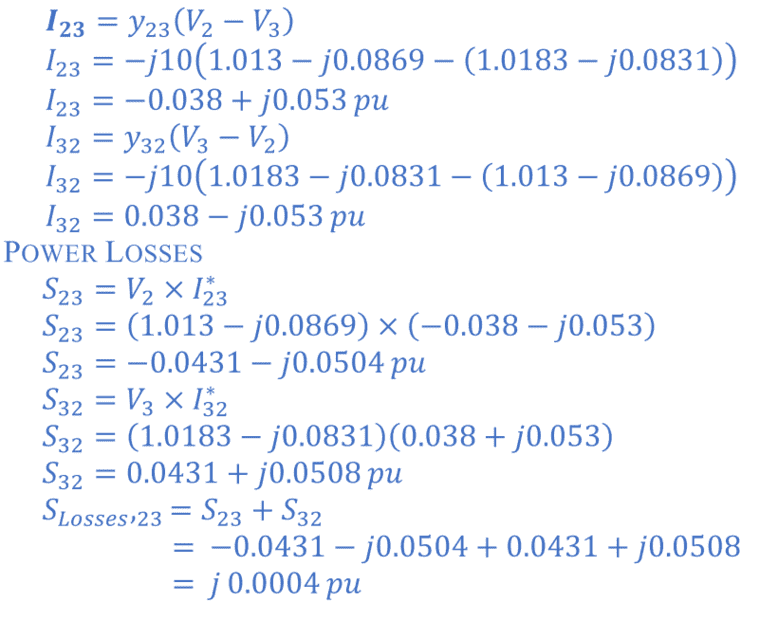# Power Flows and Losses in a line between two buses

• Engineering
• falyusuf

#### falyusuf

Homework Statement
Attached below.
Relevant Equations
Attached below.
Question:Equations:My solution:I apologize for the delay. At first glance it seemed complicated especially because Ybus = 0. in reality it is much easier because the voltages and powers are already calculated.
The calculation seems correct to me except for the losses between 2 and 3 which must be the product of V2-V3 voltage with the current and not V2 with the current conjugated as for the flow. There are not actual losses since no resistance is there but only a capacitor of 0.1 ohm reactance. That means the reactive power produced in there it is I^2*Xcap
If I23=0.038-0.053i and Iabs=0.0652 the Qloss=0.0652^2*0.1=0.0004253 [Var] p.u.
The product (V2-V3)*I23=-0.0004253 [Var] p.u. also.

Last edited: